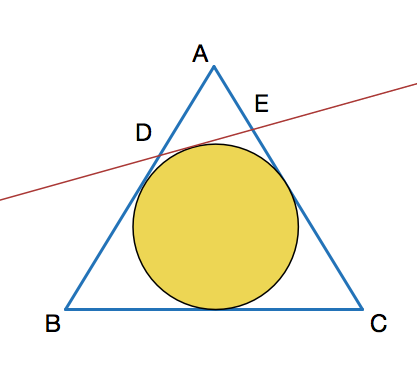# Wasn't it easy to find an equilateral triangle's side

Geometry Level 4$ABC$ is an equilateral triangle. $DE$ is the tangent to the inscribed circle with $D$ on $AB$ and $E$ on $AC$. It is given that $DE$ perpendicular to $AC$ and $AE=10$.The side length of $ABC$ can be expressed as $a+b\sqrt{c}$ where $a$ and $b$ are positive integers and $c$ is square-free positive integer; then find $a+b+c$.

×